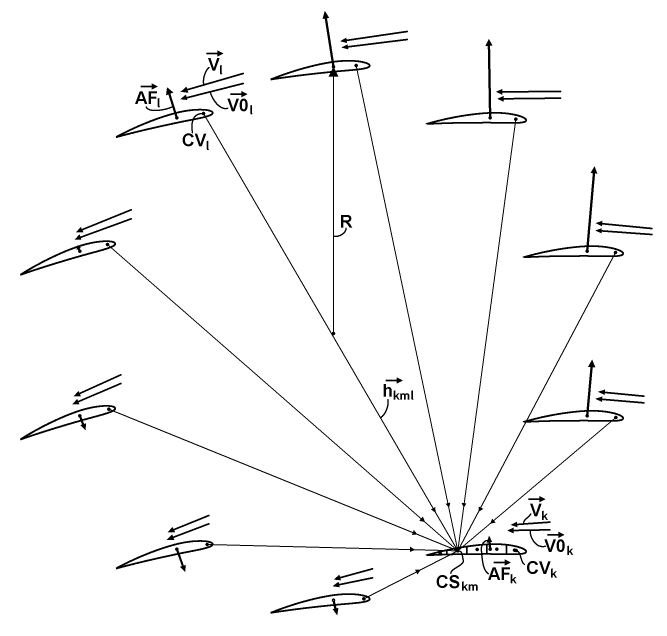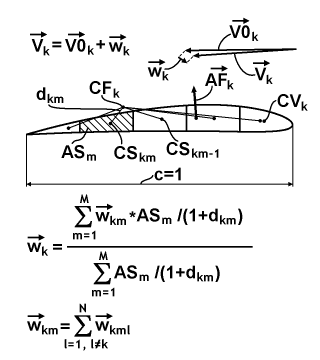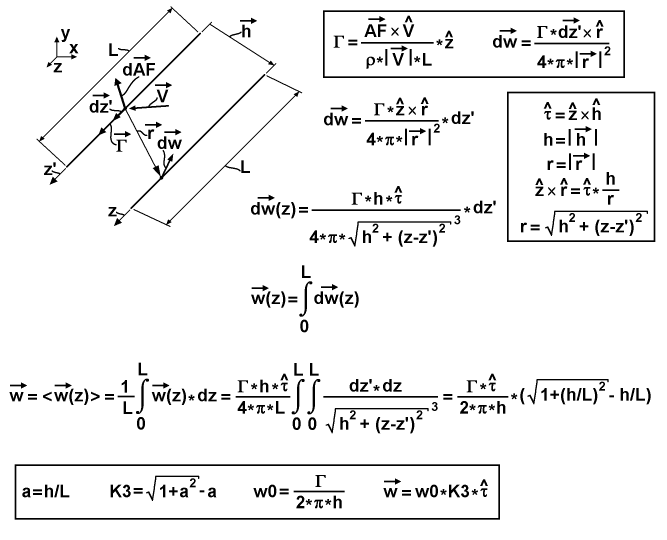﻿ Liftoplane: Interference of wings

# Interference of wings

The third aerodynamic aspect is an interference of the wings of rotor. Each wing has its own vorticity, which impacts on the base flow of other wings. This changing in the base flow of other wings changes their lift forces. And the changing of such lift forces reflects in a changing of the vorticity. And so, there are loops with mutual dependences. A final correct distribution of the airflow over wings permits the correct substitution of the aerodynamic coefficients and aggregations for obtaining a correct distribution of forces and moments.For modeling this aspect I divided each of the N wings on an M segments along their chords. It permits to define an elementary influence of all vorticities from all other (foreign) wings on these segments as it explained on the chart above. The chart depicts the wings of the rotor with a typical distribution of the aerodynamic forces AF, base flow speed vectors V0 and the actual speed vectors V. These V0 vectors already have the inflow included and these V vectors are simple individual LAS of the related wings. Each wing also has its own center of vorticity CV. The wing with the index "k" is a current destined wing. Its m-th segment receives a superposition of induced speeds from those CVs of all other wings, where the wing with the index "l" is a current source wing. The radius-vector from the l-th CV toward the center CS of the m-th segment of the k-th wing is referenced as the h-vector with the index combination "kml".The chart above represents the magnified k-th wing from the previous chart and explains how the induced speeds "w" from all segments of the wing contribute to a common induced speed. I reference it as a consolidation of the interference induced speed vector over the entire wing. For this consolidation I simple use the weighting formula, which is referenced under the shape of the wing. The weighting parameter in the formula is the ratio of the area AS of the particular segment "m" to the sum of the distance to the center of the aerodynamic force CF for the k-th wing with the chord length “c”, which is equal to 1 upon the normalization. All constituents of the consolidation formula are depicted and defined on the chart. And at bottom of the chart a simple superposition formula is represented for calculation of the induced speed on the segment itself. On the up a simple formula provides the result for the k-th V-vector.The chart above explains the calculation of the position of the center of vorticity (CVx;CVy) on a wing dependently from AoA. The center of vorticity itself isn't actually exists, since a general symmetry of vorticity distribution is too complicated for it. And so it is only approximation. At first I find a main AoA', which is equal to the AoA for the first and the fourth quadrants of the angle and equal to 180°-|AoA| for others quadrants. After it, I find a main CVx' value by the first empiric formula at bottom of the chart. And the resulted CVx will be equal to the CVx' for the first and the fourth quadrants of the angle and equal to 1-CVx' for the others. The CVy is calculated simple as y-coordinate of the camber-line of the used airfoil for the CVx coordinate. The approximation simple reflects the fact of a concentrating the vorticity toward the related leading edge of the airfoil for the high AoAs.

The center of vorticity and the counter-parted center of segment aren't points. They can be considered as linear segments with the length of wing L, with a presumed flat distribution equal to the vorticity distribution from equalized load distribution sources, having same average load as the actual wing. So there needs some specific formula, based on the correct integration on both sides for calculating the elementary value of the induced speed. The chart below explains deducing of the formula.It has in the upper-left corner a 3-d chart depicting the linear vorticity source and the linear center of the destined segment. These both linear elements are oriented in the z-direction. The y-direction is on the up and the x-direction is on the right. The z-coordinate on the sourced segment is designated as z', and for the destined segment: simple as z, for separating the different integration variables. Vector-h from the first chart is also represented there. The chart depicts an infinite small inducing action of the speed from some sub-segment on the sourced segment to some sub-segment of the destined segment. Length of the sourced sub-segment is the spatial differential dz' and length of the destined: dz. The sourced sub-segment possesses some differential value of the aerodynamic force vector-dAF, oriented perpendicular the segment and some value of the circulation vector-Γ, oriented along the segment, where both are induced by the speed vector-V of the segment. These two differential sub-segments are connected by the r-vector. The differential speed vector-dw is induced on the destined sub-segment, and it is perpendicular to the r-vector and the destined segment. Two base equations are referenced on the upper-right side of the chart in the common frame. The first is the reversed formulation of the Joukowski theorem for the case of equally loaded wing, which relates the signed scalar circulation Γ with the cross-product of the entire aerodynamic force AF and the vector-V. The second equation in the frame is the Biot-Savart law for the induced speed, which relates the differential of it with the cross-product of projection of the circulation on the vector-dz' and the vector-r. The Biot-Savart law is also expressed under the frame in the form, which reorganized to have the scalar differential dz' on its right side. The frame on the middle-right side of the chart introduces a normalized τ-vector, which is cross-product of the normalized z-vector and the normalized h-vector, and it is simple the direction of the dw-vector. Also the frame contains: a simplified notation for magnitudes h and r vectors as simple h and r; an expression for cross-product of the normalized z-vector and the normalized r-vector, which utilizes the normalized τ-vector and the ratio of h to r; and an expression for r as function of z and z'. The last two expressions are substituted in the equation for the vector-dw from the first line outside of the frame and represented at the second line as a function from z. The third line has a simple expression of an integration of the z-functional differential dw over the entire length of the sourced segment. The forth line expresses the resulted elementary induced speed vector-w as result of averaging the spatial distribution of the induced speed vector over the entire length of the destined segment. So after substitution of the first integral, there is the double integral expression, which is resulting to the simple algebraic formula upon resolving these two integrals, using tables of integrals. Finally, the frame on the bottom of the chart contains a chain form of the formula, which is friendlier for calculation. At first, there is a 3-d aspect coefficient "a" equal to the ratio of h to L. At second, there is a 3-d factor coefficient K3 expressed from the "a". At third, there is a maximal magnitude of the induced speed w0, expressed by using the entire circulation Γ and the distance between segments h. And at fourth, there is the resulted vector-w expressed as product of the w0, K3 and normalized τ-vector.The diagram above represents a data flow and definition chart for the calculation a state of the interference corrected airflow of the entire rotor. For simplify the process I use scaling of its constituents to the unitary wing's chord, the unitary air density and the unitary wing's area. But the airspeed is kept in the absolute units. So at first, there are scaled definitions, where each scaled component has a ' sign, including the chord itself, the radius of rotor, the length of wings, the distances between source and destination segments, the formulation of aerodynamic force and the formulation of circulation, where for last is used the two-dimensional scalar version of the cross-product. At second, there is an input setup of the known values, which includes the Reynolds number Re0=c/ν, normalized on speed, the common and constant pivot’s and segments’ properties and the distributions of wings positions, pitches and base flows. And the setup is finalized by calculating the spatial distribution of centers of segments and setting the distribution of the initial airspeed equal to the respective base flow. After the setup, a main cycle for the updating result of each wing begins. It includes calculating of the speed magnitude, the Reynolds number, the AoA, querying of the aerodynamic coefficients and aggregations, referenced there as Polar, calculating the scaled aerodynamic force and circulation, calculating the center of vorticity relative the chord and reposition CF and CV relative the rotor's origin, which is center of the rotor. After 2N cycles of this updating the completed result passes to the output. In other case, the updating cycle continues for updating the distribution of airspeeds themselves, beginning from a walkthrough of destined wings. This walkthrough begins from a walkthrough of segments, which begins from the reset of related induced speed to zero and continues with a walkthrough of foreign sourced wings. The last walkthrough performs an incremental update of the current segment, which includes: calculating the radius-vector from current CV to current CS; calculating the direction of induced speed, calculating the 3-d aspect coefficient, calculating the 3-d factor coefficient, calculating the maximal magnitude of induced speed, calculating the induced speed itself and accumulating the induced speed on the current segment. After it, the last walkthrough continues. After the finalizing of the last walkthrough, it returns to continue the walkthrough for next segment. After the finalizing of the walkthrough of segments, there begins a consolidating stream for the current destined wing. This consolidation uses the formulation referenced before on the second chart (I used there a tensorial notation for compactness), which is finalizing by updating the airspeed of the current destined wing. The consolidation returns to continue the walkthrough for next destined wing. The walkthrough of destined wings is finishing by initiation of the next main cycle for updating of the result.